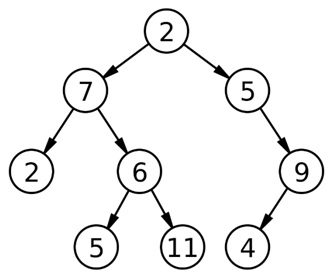Q:

# Reverse Level Order Traversal

Write a program to print Reverse Level Order Traversal of a binary tree.

Example:```    Basic level order traversal:
2
7 5
2 6 9
5 11 4

Reverse Level order traversal:
5 11 4
2 6 9
7 5
2```

Of course the solution is quite like the level order traversal just a little bit of modification.

Algorithm:

FUNCTION reverseLevelOrder (root): prints reverse Level order traversal of the tree

Prerequisite: Queue q, Stack s

```FUNCTION reverseLevelOrder(root)// root of the tree
1.  Declare a TreeNode type variable temp;
2.  Declare qto store nodes //creating a queue
3.  Declare sfor reversal
4.  IF (!root)   //root is null
return;

5.  EnQueue(q,root); // EnQueue the root in the queue

6.  while(q is not empty){
temp=DeQueue(q);  //Dequeue
s.push(temp->data); //push temp->data to stack
IF(temp->right)//this is different from ordinary level-order
EnQueue(q,temp->right); // if right child exists EnQueue
END IF
IF(temp->left)
EnQueue(q,temp->left); // if left child exists EnQueue
END IF
END While
7.  While(s is not empty)
Pop and print
END While
8.  DeleteQueue(q);
9.  DeleteStack(s);
```

Example with explanation:N.B: The nodes are represented by their respective values.

For the above example:

```In the main we call reverseLevelOrder (2)
------------------------------------------------------------

reverseLevelOrder (2)
q.push(2)//EnQueue the root
------------------------------------------------------------

1st iteration:
q is not empty
temp= q.front()=2
q.pop()
Queue status:
Empty Queue
s.push(temp->data) //2
2->right not NULL
q.push(2->right) //q.push(5)
2->left not NULL
q.push(2->left)//q.push(7)
Queue status:
5 7
------------------------------------------------------------

2nd iteration:
q is not empty
temp= q.front()=5
q.pop()
Queue status:
7
s.push(temp->data) //5
5->right not NULL
q.push(5->right) //q.push(9)
5->leftNULL
Queue status:
7 9
------------------------------------------------------------

3rd iteration:
q is not empty
temp= q.front()=7
q.pop()
Queue status:
9
s.push(temp->data) //7
7->right not NULL
q.push(7->right) //q.push(6)
7->left not NULL
q.push(7->left) //q.push(2)
Queue status:
9 6 2
------------------------------------------------------------

4th iteration:
q is not empty
temp= q.front()=9
q.pop()
Queue status:
6 2
s.push(temp->data) //9
9->rightNULL
9->left not NULL
q.push(9->left) //q.push(4)
Queue status:
6 2 4
------------------------------------------------------------

5th iteration:
q is not empty
temp= q.front()=6
q.pop()
Queue status:
2 4
s.push(temp->data) //6
6->right not NULL
q.push(6->right) //q.push(11)
9->left not NULL
q.push(6->left) //q.push(5)
Queue status:
2 4 11 5
------------------------------------------------------------

6th iteration:
q is not empty
temp= q.front()=2
q.pop()
Queue status:
4 11 5
s.push(temp->data) //2
2->rightNULL
2->left  NULL
Queue status:
4 11 5
------------------------------------------------------------

7th iteration:
q is not empty
temp= q.front()=4
q.pop()
Queue status:
11 5
s.push(temp->data) //4
4->right NULL
4->left   NULL
Queue status:
11 5
------------------------------------------------------------

8th iteration:
q is not empty
temp= q.front()=11
q.pop()
Queue status:
5
s.push(temp->data) //11
11->right NULL
11->left   NULL
Queue status:
5
------------------------------------------------------------

9th iteration:
q is not empty
temp= q.front()=5
q.pop()
Queue status:
Empty queue
s.push(temp->data) //5
5->right NULL
5->left   NULL
Queue status:
Empty Queue
------------------------------------------------------------

10th iteration:
Empty Queue

Final stack status:
5
11
4
2
6
9
7
5
2

Pop and print:
Output:
5 11 4 2 6 9 7 5 2

```

C++ implementation:

``````#include <bits/stdc++.h>
using namespace std;

class Node{    // tree node is defined
public:
int data;
Node *left;
Node *right;
};

void reverseLevelOrder(Node *root)
{
Node* temp;
stack<int> s;
queue<Node*> q;
q.push(root);
while(!q.empty()){
temp=q.front();
q.pop();
s.push(temp->data);
if(temp->right)
q.push(temp->right);
if(temp->left)
q.push(temp->left);
}
while(!s.empty()){
cout<<s.top()<<" ";
s.pop();
}
}

Node* newnode(int data)  // creating new node
{
Node* node = (Node*)malloc(sizeof(Node));
node->data = data;
node->left = NULL;
node->right = NULL;
return(node);
}

int main() {
//**same tree is builted as shown in example**
Node *root=newnode(2);
root->left= newnode(7);
root->right= newnode(5);
root->right->right=newnode(9);
root->right->right->left=newnode(4);
root->left->left=newnode(2);
root->left->right=newnode(6);
root->left->right->left=newnode(5);
root->left->right->right=newnode(11);

cout<<"Reverse Level Order traversal of binary tree is :"<<endl;
reverseLevelOrder(root);

return 0;
}
``````

Output

```Reverse Level Order traversal of binary tree is :
5 11 4 2 6 9 7 5 2 ```